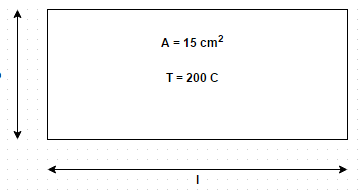# Test: Laminar Flow

## 10 Questions MCQ Test | Test: Laminar Flow

Description
Attempt Test: Laminar Flow | 10 questions in 10 minutes | Mock test for Chemical Engineering preparation | Free important questions MCQ to study for Chemical Engineering Exam | Download free PDF with solutions
QUESTION: 1

### The Nusselt number is related to Reynolds number in laminar and turbulent flows respectively as

Solution:

Nusselt number = h l/k and Reynolds number = p V l/µ.

QUESTION: 2

### A hot plate of 15 cm2 area maintained at 200 degree celsius is exposed to still air at 30 degree Celsius temperature. When the smaller side of the plate is held vertical, convective heat transfer rate is 15 per cent higher than bigger side of the plate is held vertical. Find size of the plate. The appropriate correlation for the convection coefficient isNu = 0.60 (Gr Pr) 0.25

Solution:

= 200 + 30/2 = 115 degree celsius. Pr = µ c/k, Gr = bβ g d t/µ 2 and Nu = h b/k.

QUESTION: 3

### Which of the following is true for laminar flow?

Solution:

The product G r is often referred to as Rayleigh number, and its value sets the criterion of laminar character of flow.

QUESTION: 4

A horizontal heated plate at 200 degree Celsius and facing upwards has been placed in still air at 20 degree Celsius. If the plate measures 1.25 m by 1 m, make calculations for the heat loss by natural convection. The convective film coefficient for free convection is given by the following empirical relation
h = 0.32 (α) 0.25 W/m2 K
Where α is the mean film temperature in degrees kelvin

Solution:

α = 273 + 200 + 20/2 = 383 degree Celsius. Therefore, rate of heat loss = h A d t = 3006 W.

QUESTION: 5

For laminar flow, Reynolds number must not be less than

Solution:

It should not be less than 40000.

QUESTION: 6

For laminar flow, Prandtl number must be more than

Solution:

It must be more than 0.6. It is indicative of the relative ability of the fluid to diffuse momentum and internal energy by molecular mechanisms.

QUESTION: 7

Air at atmospheric pressure and 20 degree Celsius flows with 6 m/s velocity through main trunck duct of air conditioning system. The duct is rectangular in cross-section and measures 40 cm by 80 cm. Determine heat loss per meter length of duct corresponding to unit temperature difference. The relevant thermos-physical properties of air are
v = 15 * 10 -6 m 2/s
α = 7.7 * 10 -2 m2/hr
k = 0.026 W/m degree

Solution:

Q = h A d t, Area = 2 (a + b) (1) = 2.4 m2, h = 365.34 k/l.

QUESTION: 8

The local film coefficient for laminar flow past a flat plate may be obtained from the correlation

Solution:

Fluid properties are evaluated at mean film temperature.

QUESTION: 9

For a plate of length l, an average value of Nusselt number is given by

Solution:

For a plate of length l, an average value of Nusselt number or convection coefficient may be obtained by integration.

QUESTION: 10

The correlation for liquid metal is given by

Solution:

It is valid for Pr less than or equal to 0.05.Use Code STAYHOME200 and get INR 200 additional OFF Use Coupon Code